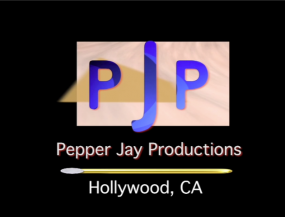# Influencers Unite – Keynote Speakers Coalition presented by ezWay Broadcasting

<p< < p=””>

ezWay Broadcasting presents Influencers Unite – Keynote Speakers Col coverage by Actors Reporter, a channel on the Actors Podcast Network, a Pepper Jay Production. <p< < p=””>

Dante Obligacion on the red carpet for Actors Reporter at the Influencers Unite – Keynote Speakers Coalition.

<p< < p=””> </p< <> </p< <></p< <>

<p< < p=””>

Influencers Unite
<p< < p=””> </p< <>

<p< < p=””>
eZWay Events presents Influencers Unite in conjunction with West Coast Speakers Coalition. Event live stream coverage by Voice America TV in Dana Point OC <p< < p=””>

Top notch speakers / Professionally catered / Media covered Red Carpet <p< < p=””>

<p< < p=””>

<p< < p=””>center> <p< < p=””> </p< <>

<p< < p=””> <p< < p=””>

Featured Speakers: Pete Vargas, Emily Letran <p< < p=””> </p< <>

Keynote Speakers Coalition Panel: Allison Hildebrandt Larsen, David T. Fagan, Tayna Brown, Rachelle Caco, Dan Clark, Eric Zuley, Jeff Spenard, James Dentley <p< < p=””> </p< <>

Â eZWay Media Panel: Lester Speight, Reatha Grey, Summer Helene, Brad Lewis, Count and Countess Anjelika Kaufman and John Pierre Joyce <p< < p=””> </p< <>

Celebrity Hollywood Speaker: Marcello Thedford <p< < p=””> </p< <>

Special Honoree James Zuley
Award presented by Eric Zuley, Carmelita Pittman and (ret) Senator Pasqual <p< < p=””>

<p< < p=””>

Performers <p< < p=””> </p< <>

Evan Disney
Scorpio <p< < p=””>

<p< < p=””>

Awards Presented:

Keynote Speakers Coalition founder Allison Hildebrandt Larsen presented awards to:

James Dentley
Eric Zuley
David T. Fagan <p< < p=””> </p< <>

Eric Zuley
Carmelita Pittman
Senator (Ret) Pasqual <p< < p=””> </p< <>

Presented the Angel Award and Salute to America certificate to James Zuley <p< < p=””> </p< <>

<p< < p=””>

Event catered by: Celebrity Pizza Guy and Akioni

Event produced by: http://ezwaymedia.com
in conjunction with http://speakerscoalition.com & http://voiceamerica.com

<p< < p=””><p< < p=””>

<p< < p=””> <p< < p=””> </p< <></p< <></p< <></p< <></p< <></p< <></p< <></p< <></p< <></p< <></p< <></p< <></p< <><p< < p=””>

<p< < p=””> <p< < p=””><p< < p=””>